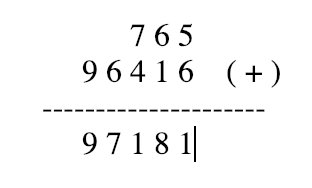Showing posts with label Addition of 2 Linked List. Show all posts
Showing posts with label Addition of 2 Linked List. Show all postsFor example:

val_1[] = {       7, 6, 5 }
val_2[] = { 9, 6, 4, 1, 6 }

then the output should be {9, 7, 1, 8, 1} basically its addition of 1st and 2nd linked lists as same normal 2 numbers addition.

Let's see simple java code to create linked lists using input array and to get the addition of 2 linked list in 3rd linked list.

```public class SumOfTwoLL {

class NODE {

int data;
NODE next;

public NODE(int data) {
this.data = data;
this.next = null;
}
}

public static void main(String[] args) {

SumOfTwoLL obj = new SumOfTwoLL();

int val_1[] = new int[] {       7, 6, 5 };
int val_2[] = new int[] { 9, 6, 4, 1, 6 };

// Create linked list out of arrays

// Check no. of nodes in both Linked list
int t1 = obj.noOfNodes(first);
int t2 = obj.noOfNodes(second);

NODE third = obj.addTwoLL(first, second, 0);

obj.print(third);

}

// Method to get sum of 2 Linked List
private NODE addTwoLL(NODE first, NODE second, int cnt) {

if(first != null && second != null){

// Recursive call moving to last node
NODE node = addTwoLL(first.next, second.next, cnt+1);

// Calculating sum from last 2 values
int val = (first.data + second.data) +node.data;

int carry = val/10;
int sum = val%10;

// If carry present or not the first node in list then create a node with carry value
if(cnt != 0 || carry > 0) {

NODE carryNode = new NODE(carry);
node.data = sum;
carryNode.next = node;
return carryNode;

}else {
// Assign final sum to the previous cycle carry node
node.data = sum;
return node;
}
}
// Create last carry node with zero
return new NODE(0);
}

NODE tmp = new NODE(0);
tmp.next = ll;
ll = tmp;
}
return ll;
}

private int noOfNodes(NODE ll){
int count = 0;
while(ll !=  null){
count ++;
ll = ll.next;
}
return count;

}

NODE start = null;
for (int i : val) {
NODE tmp = new NODE(i);
if (start == null) {
start = tmp;
} else {
NODE mover = start;
while (mover.next != null) {
mover = mover.next;
}
mover.next = tmp;
}
}
return start;
}

public void print(NODE tmp){
while(tmp != null ){
System.out.print(tmp.data +", ");
tmp = tmp.next;
}
}
}
```

OUTPUT:

```9, 7, 1, 8, 1,
```